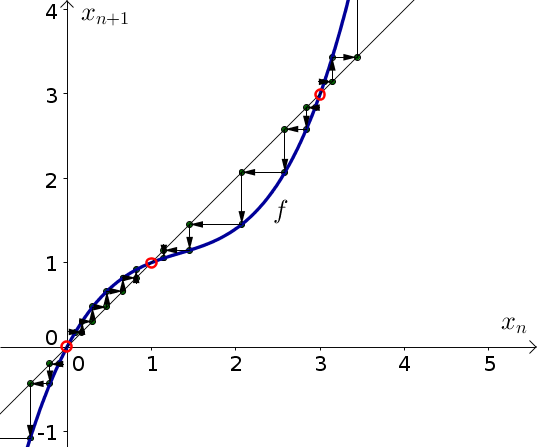# Math Insight

### Image: Discrete dynamical system example function 1, with cobwebbingThe function $x_{n+1}=f(x_n)=x_n + 0.3x_n (x_n - 1) (x_n - 3)$ is plotted in blue along with the diagonal line $x_{n+1}=x_n$. Equilibria of the dynamical system are illustrated by the red circles at the points where the two graphs intersect. Cobwebbing is indicated by the arrows.

Source image file: discrete_dynamical_system_example_function_1_cobweb.ggb
Source image type: Geogebra